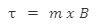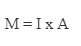• Call Now

1800-102-2727•

# Magnetic Moment

The magnetic moment, also known as the magnetic dipole moment, is a measurement of an object's inclination to align with a magnetic field.
"The magnetic direction and strength of a magnet or other item that creates a magnetic field are explained as a magnetic moment."

The magnetic moment is measured as a vector quantity. The items have a tendency to align themselves such that the magnetic moment vector is parallel to the magnetic field lines.
The magnetic moment of a magnet points from the south to the north pole. A magnet's magnetic field is accurately proportional to its magnetic moment.

Formula of Magnetic Dipole Moment: A magnetic moment is a vector quantity. It connects an object's torque to a magnetic field. This is expressed mathematically as:Where,

• m = magnetic moment
• B = external magnetic field
• τ = torque acting on the dipole

## How is a magnetic moment created?

The magnetic moment is created using one of two methods:

• Spin Angular Momentum
• The Electric Charge’s motion

## Calculation of Magnetic Moment

Magnetometers are equipment used to calculate magnetic moments. However, not all magnetometers are gauged to detect the magnetic moment. Some of these sensors just measure magnetic fields, and the magnetic moment is calculated based on the observed magnetic field.

Unit of Magnetic Moment: The magnetic moment is defined in the articulation of the current loop as the product of the current flowing and the area,• As a result, Amp-m2 articulates the unit imparting this definition.
• It is also possible to express it in terms of moment and torque. As a result, the torque is measured in Joules (J) and the magnetic field in tesla (T). The unit is JT-1.
• As a result, these two units are comparable and are supplied by 1 Amp-m2 = 1 JT-1.

## Magnetic Dipole Moment

A Magnetic Dipole is made up of two opposing poles of equal strength separated by a small distance. Magnetic dipoles include a bar magnet, a compass needle, and so on. We will demonstrate how a current loop functions as a magnetic dipole.
The product of pole strength and the distance between the two poles is defined as magnetic dipole moment. The magnet length is defined as the distance between the two poles of a magnetic or magnetic dipole and is expressed as the 2.
If m is the power of any magnetic pole, the magnetic dipole moment of the magnet is represented by M, which is written as:
M = m/2

The magnetic dipole moment is a vector with a direction from the magnet's south to its north pole. The force on a magnetic dipole is due to both poles of the magnet, and we study the magnetic dipole of a bar magnet and assume that the magnet is kept in an undamaged magnetic field B. In such case, the force on the individual poles is coupled as:

• mB, which is associated with the magnetic field B, equals force on the North pole.
• mB, which is the inverse of magnetic field B = Force on the South pole.

These forces are identical in magnitude but opposite in direction. They form a parallel couple that rotates the magnet in a clockwise direction. It produces a net torque on the magnet due to the individual forces in a couple. Hence, torque acts on the bar magnet.

τ is the Moment of the couple.

τ = mB × 2L sin θ

As a result of the preceding explanation, where θ is the angle between the magnetic field and the magnet.

M = m x 2L

As a result, the magnetic dipole moment is articulated by:

ττ = MB sin θ

In vector form, it can be paraphrased as:

τ = M × B

The magnetic dipole force must be expressed in this manner.Talk to our expert
Resend OTP Timer =
By submitting up, I agree to receive all the Whatsapp communication on my registered number and Aakash terms and conditions and privacy policy